Example Questions

2 Next →

Example Question #11 : Basic Squaring / Square Roots

Simplify: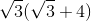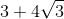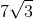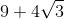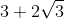Explanation:

To simplify the problem, just distribute the radical to each term in the parentheses.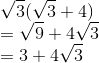Example Question #12 : Basic Squaring / Square Roots

Simplify: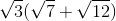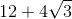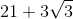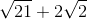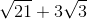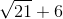Explanation:

To simplify the problem, just distribute the radical to each term in the parentheses.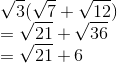Example Question #13 : Basic Squaring / Square Roots

Simplify: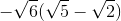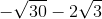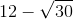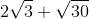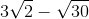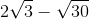Explanation:

To simplify the problem, just distribute the radical to each term in the parentheses.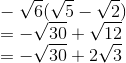Example Question #21 : Basic Squaring / Square Roots

Simplify: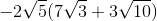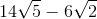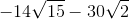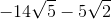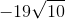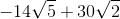Explanation:

To simplify the problem, just distribute the radical to each term in the parentheses.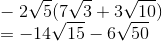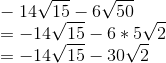Example Question #22 : Basic Squaring / Square Roots

Simplify: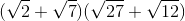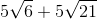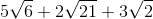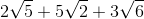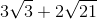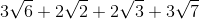Explanation:Let's simplify the right parentheses.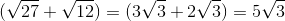Now we can distribute the radical to each term in the parentheses.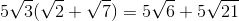2 Next →